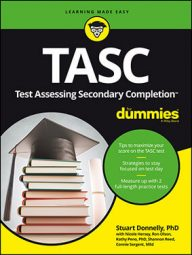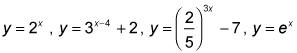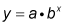##### TASC For DummiesYou will probably encounter questions on the TASC Math exam that involve exponential functions. If the problems appear in the form of a graph or a table, the following instructions will help you navigate through them.

An exponential function is when the independent variable is in the exponent of a constant. The base of the function must be greater than 0 and not equal to 1. Some examples of exponential functions are:If the base of the exponential function is a fraction, then the graph falls rapidly to the right, as shown here.

Looking for these general shapes when given a graph will indicate whether the graph represents an exponential function.

If you're given a table, to determine whether the function is exponential, check if there's a common multiple difference, meaning you can multiply each of the y-values by a number to get to the next y-value. This indicates that the function is exponential and, in fact, that that number is your base.

To write an equation for an exponential function, follow these steps:

1. Find the common multiple difference (this is your base, b).
2. Find your y-intercept—this is the coefficient of your exponential function (a).
3. Substitute your values for a and b into the general form of an exponential:For example:

First find whether there's a common multiple difference. It's important to note that the x-values are evenly spaced, which allows the common multiple to be identifiable.

You now know that your base is 2. Looking for your y-intercept, you see it's (0, 3), so a = 3. Now substitute these values into

Stuart Donnelly, PhD, was awarded a PhD in mathematics from Oxford University. He has prepared students for the TASC test and GED Test for the past two decades.

Stuart Donnelly, PhD, earned his doctorate in mathe-matics from Oxford University at the age of 25. Since then, he has established successful tutoring services in both Hong Kong and the United States and is considered by leading educators to be one of the most experienced and qualified private tutors in the country.

Stuart Donnelly, PhD, was awarded a PhD in mathematics from Oxford University. He has prepared students for the TASC test and GED Test for the past two decades.

Stuart Donnelly, PhD, was awarded a PhD in mathematics from Oxford University. He has prepared students for the TASC test and GED Test for the past two decades.

Sandra Luna McCune, PhD, is professor emeritus and a former Regents professor at Stephen F. Austin State University. She's now a full-time author. Shannon Reed, MA, MFA, is a visiting lecturer at the University of Pittsburgh, where she teaches composition, creative writing, and business writing.

Stuart Donnelly, PhD, was awarded a PhD in mathematics from Oxford University. He has prepared students for the TASC test and GED Test for the past two decades.